##### Algebra I For Dummies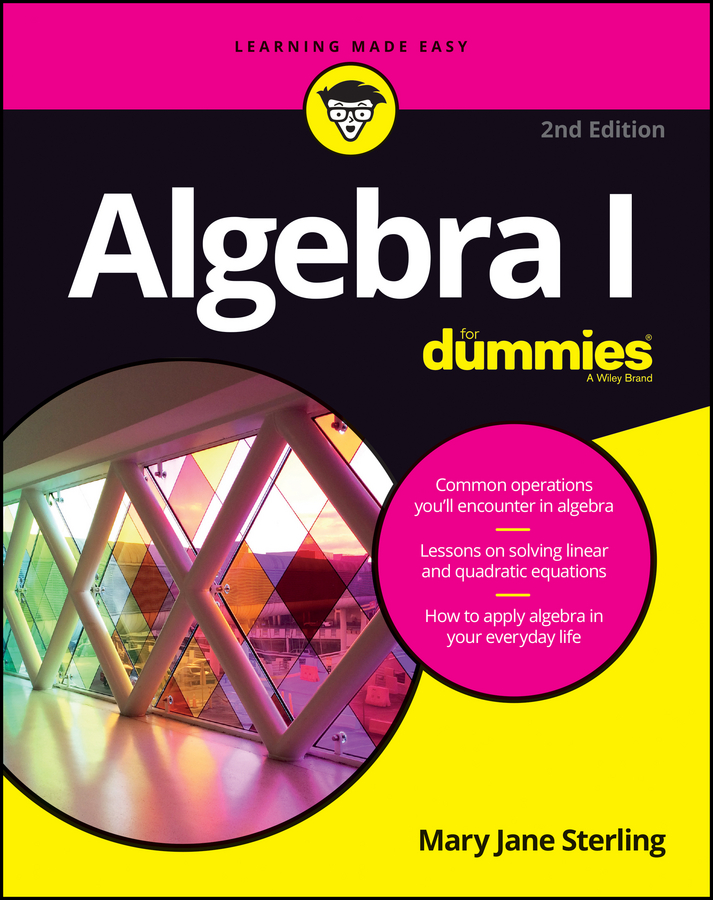Ratios and proportions are an important part of the study of math, science, and business. A ratio can be written as a fraction, and a proportion is a statement that two ratios are equal. The properties of proportions are most helpful when solving problems whose equations use proportions.

First, consider the properties of proportions. Given the proportion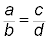the following is true:

• The cross products are equal: ad = bc.

• The reciprocals are equal: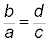• The following fractions can be reduced as usual.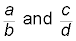The fractions in the following can be reduced vertically or horizontally by removing common factors:So, if a = ef and c = eg, then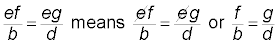And if b = jk and d = jm, then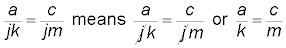These properties are used to work with the golden ratio, the relationship found in the golden rectangle. The golden rectangle is believed to be the most aesthetically pleasing form and appears in masterpieces such as the Mona Lisa and in other art and architecture for centuries.

In Euclids Elements, you find one of the first written definitions of this golden ratio: “A straight line is said to have been cut into extreme and mean ratio when, as the whole line is to the greater segment, so is the greater to the lesser.” What, is that Greek to you?

Consider a segment divided into two parts, a and b, representing the golden ratio.

The segment a is 1 unit long, and the segment a + b is about 1.618 units long, an approximation of the golden ratio. So, the ratio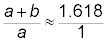The approximate symbol is used here because the golden ratio is an irrational number and has a decimal that never ends or repeats. What’s so special about this particular ratio?

Back to Euclid’s definition, the “as the whole line is to the greater segment” (the length 1.618 is to 1) “so is the greater to the lesser” (the length 1 is to 0.618). The ratio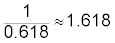The proportion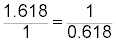To find the exact value of the golden ratio, consider the proportion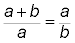If the length of a is 1 unit, then the proportion becomes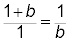Use the cross-product property to get (1 + b)b = 1 or b + b2 = 1. In the standard form of a quadratic equation in b, you have b2 + b – 1 = 0. To solve for b, you need the quadratic formula: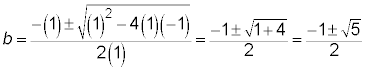You consider only the sum in the numerator, because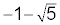is a negative number and can’t represent the length of the segment. So let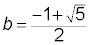Now compute a + b by replacing the a with 1 and the b with the solution from the quadratic formula, and you have: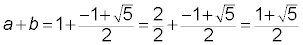The value of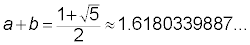or the golden ratio.